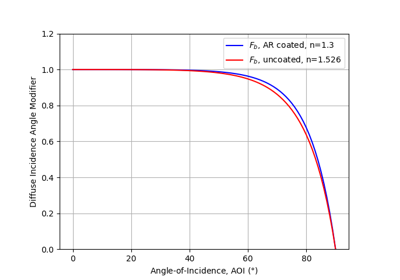# pvlib.iam.physical¶

pvlib.iam.physical(aoi, n=1.526, K=4.0, L=0.002)[source]

Determine the incidence angle modifier using refractive index n, extinction coefficient K, and glazing thickness L.

iam.physical calculates the incidence angle modifier as described in , Section 3. The calculation is based on a physical model of absorbtion and transmission through a transparent cover.

Parameters: aoi (numeric) – The angle of incidence between the module normal vector and the sun-beam vector in degrees. Angles of 0 are replaced with 1e-06 to ensure non-nan results. Angles of nan will result in nan. n (numeric, default 1.526) – The effective index of refraction (unitless). Reference  indicates that a value of 1.526 is acceptable for glass. K (numeric, default 4.0) – The glazing extinction coefficient in units of 1/meters. Reference  indicates that a value of 4 is reasonable for “water white” glass. L (numeric, default 0.002) – The glazing thickness in units of meters. Reference  indicates that 0.002 meters (2 mm) is reasonable for most glass-covered PV panels. iam (numeric) – The incident angle modifier

Notes

The pvlib python authors believe that Eqn. 14 in  is incorrect, which presents $$\theta_{r} = \arcsin(n \sin(AOI))$$. Here, $$\theta_{r} = \arcsin(1/n \times \sin(AOI))$$

References

  (1, 2, 3, 4) W. De Soto et al., “Improvement and validation of a model for photovoltaic array performance”, Solar Energy, vol 80, pp. 78-88, 2006.
  Duffie, John A. & Beckman, William A.. (2006). Solar Engineering of Thermal Processes, third edition. [Books24x7 version] Available from http://common.books24x7.com/toc.aspx?bookid=17160.

## Examples using pvlib.iam.physical¶Diffuse IAM Calculation